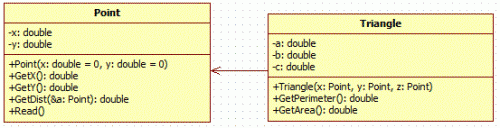favorite We need a little bit of your help to keep things running, click on this banner to learn more
Competitions

# Perimeter and area of triangle

The real numbers `x1`, `y1`, `x2`, `y2`, `x3`, `y3` - the coordinates of triangle vertices are given. Find the perimeter and the area of a triangle.#### Input

In one line the coordinates of triangle vertices are given: 6 numbers `x1`, `y1`, `x2`, `y2`, `x3`, `y3` that do not exceed 100 by absolute value.

#### Output

Print in one line the perimeter and the area of a triangle. Print the answer with 4 decimal digits.

Time limit 1 seconds
Memory limit 122.17 MiB
Input example #1
```3 2 7 6.5 10 1
```
Output example #1
```19.3568 17.7500
```
Source SFE-2010 Variant 25